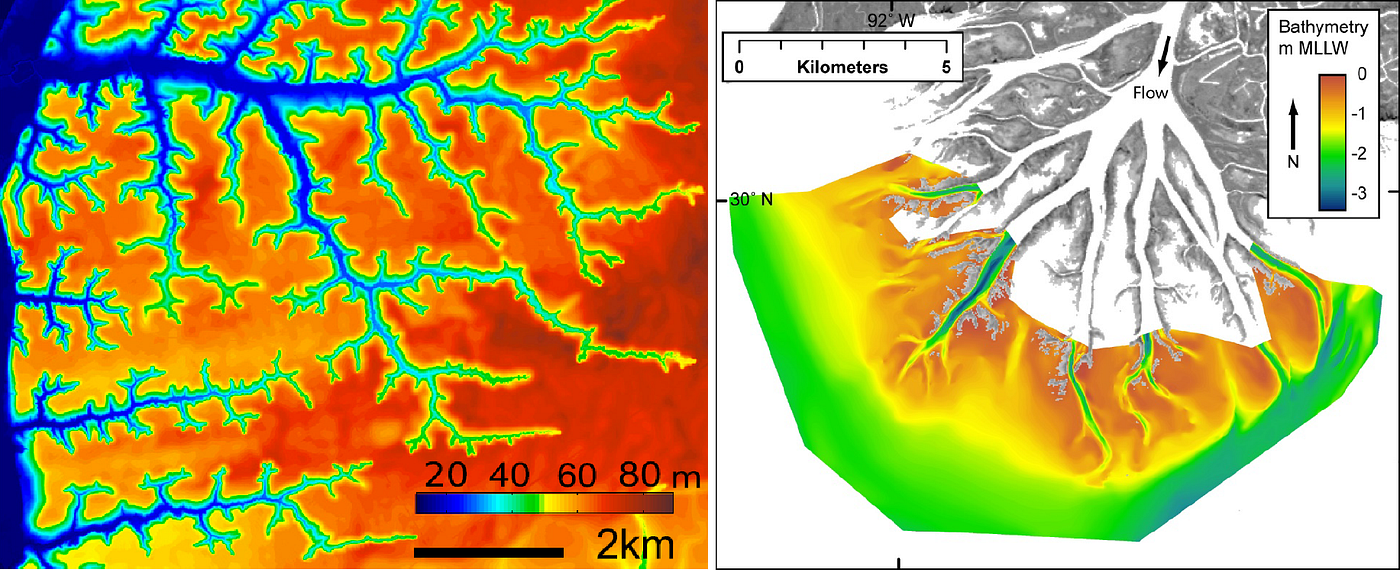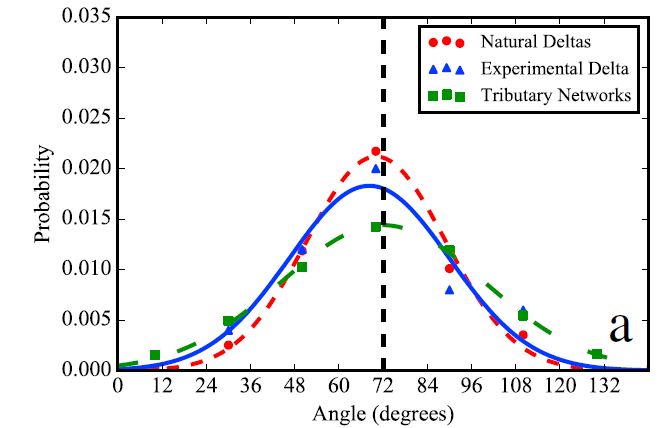# Branching angles of river channels are similar, whether they join or split.The left picture shows a digital elevation model of the channel network at Apalachicola Bluffs and Ravines Preserve, near Bristol, Florida. Here, the network branches where two channels flow into one at a confluence. The colors refer to an elevation above sea level. This figure is published here. The right picture shows the Wax Lake Delta near Berwick, Louisiana. Here, the colors show elevations relative to low tide. This is a distributary network, where most of the branches form where one channel splits into two. This figure is published here.The average branching angle of channel networks approaches 72˚ in many cases. The red circles, blue triangles, and green squares show a histogram of measured angles. The colored lines show normal distributions (bell curves) fit to each dataset. In each case, the average angle is close to the theoretical prediction of 72˚.

--

--